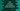# Compare two numbers using compareTo method in Dart### Compare two numbers using compareTo method in Dart :

In this dart tutorial, we will learn how to compare two numbers using compareTo methods. We know that numbers in dart can be categorized into two types: integer and doubles. Dart has one inbuilt method compareTo to compare two different integer or double variables. We can define this method as below :

``````int compareTo (
num other
)``````
1. It takes one number variable as argument.
2. The return value is 0 if both numbers are equal, a negative number if the current number is less than the number passing as an argument and a positive number if the current number is greater than the argument number.

One thing we should remember that -0.0 is less than 0.0. Let’s try to implement this with an example :

``````import 'dart:io';

main(){
stdout.writeln("Enter first number : ");

stdout.writeln("Enter second number : ");

if(firstNumber.compareTo(secondNumber) == 0){
stdout.writeln("\$firstNumber is equal to \$secondNumber");
}else if(firstNumber.compareTo(secondNumber) < 0){
stdout.writeln("\$firstNumber is smaller than \$secondNumber");
}else{
stdout.writeln("\$firstNumber is greater than \$secondNumber");
}

}``````

### Sample Output :

``````Enter first number :
5
Enter second number :
2
5 is greater than 2

Enter first number :
3
Enter second number :
3
3 is equal to 3

Enter first number :
3
Enter second number :
4
3 is smaller than 4``````